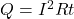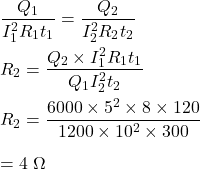## The heat developed in an electric wire varies jointly as the wires resistance, the time the current flows, & the square of the current.

Question

The heat developed in an electric wire varies jointly as the wires resistance, the time the current flows, & the square of the current. In two minutes a current of 5 amps develops 1,200 heat units in a wire with a current of 10 amps in 5 minutes?

in progress 0
2 months 2021-07-29T17:37:07+00:00 1 Answers 2 views 0

4 ohms

Step-by-step explanation:

The question is “In two minutes a current of 5 amps develops 1,200 heat units in a wire of 8 ohms resistance. What resistance does a similar wire have, which develops 6,000 heat units with a current of 10 amps in 5 minutes?”

Current, I₁ = 5 A

Heat, Q₁ = 1200 units

Resistance, R₁ = 8 ohms

Time, t₁ = 2 min = 120 s

New heat, Q₂ = 6000 units

Current, I₂ = 10 A

New time, t₂ = 5 min = 300 s

We need to find the new resistance.

Heat developed is given by :So, the new resistance is 4 ohms.